The Gold MPA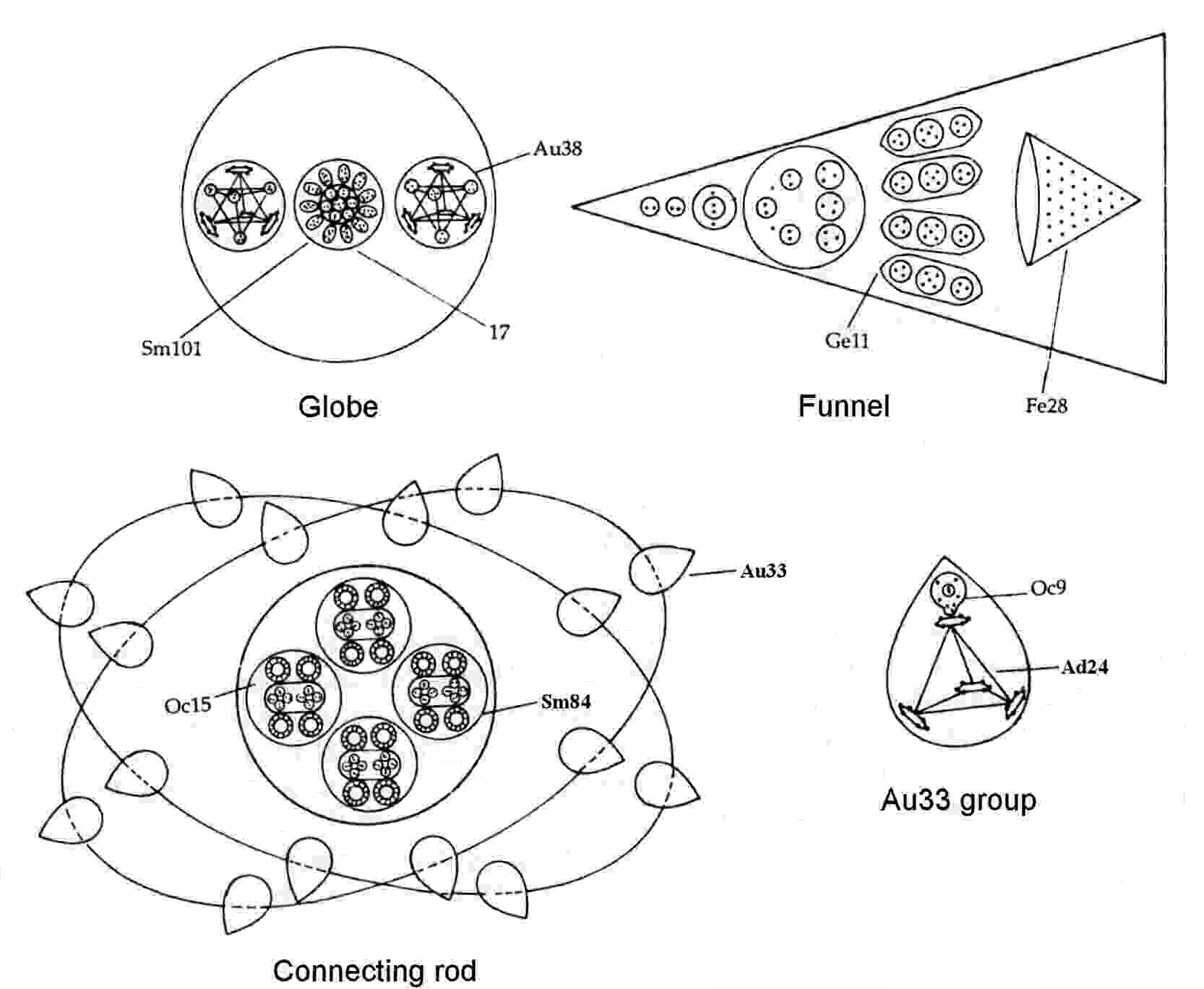The MPA of gold is shaped like a dumb-bell. Its connecting rod consists of four Sm84 groups1 around which sixteen Au33 groups revolve in two inclined orbits, eight to each orbit. The Sm84 group consists of two clusters of four triplets of UPAs and four Oc15 rings like that in the MPA of so-called "Occultum" (this is the MPA of the He3 isotope2). The Au33 group consists of a tetrahedral Ad24 group and a balloon-shaped Oc9 group similar to that in the Occultum MPA. Each of the globes at the ends of the connecting rod is made up of an Sm101 group and two Au38 groups. The Sm101 consists of a ring of twelve I7 groups arranged symmetrically about an mNe5 group, which is at the centre of another ring of six duads of UPAs. The Au38 consists of an Ad24 group that intersects a tetrahedral array of two Be4 groups and two hydrogen triplets. Each of the twenty-four funnels contains a Cl25 group, four Ge11 groups and a Fe28 cone containing twenty-eight UPAs.

Gold MPA = (4Sm84 + 16Au33) + 2(Sm101 + 2Au38) + 24(Cl25 + 4Ge11 + Fe28).

The MPA of gold is formed from two Au197 nuclei, which provide 3546 subquarks — exactly the same as the number of UPAs. The sceptic has to dismiss this precise agreement between theory and observation as a coincidence. It is highly implausible given the fact that the Au197 isotope is the only stable nuclide of gold, whilst the 'International List' of 1905, the scientific list of atomic weights to which Besant & Leadbeater said they referred to check their work, specified the atomic weight of gold as 195.74. This means that the number weight, defined generally for MPAs as:

number weight = UPA population/18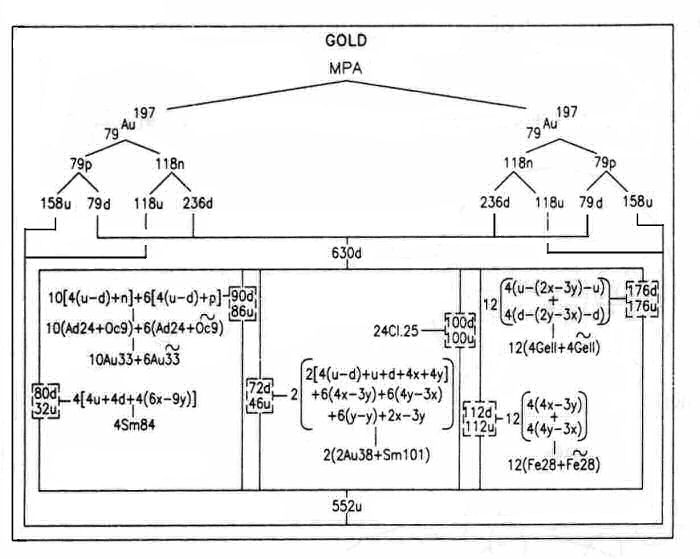would have been in the case of gold either 195 or 196 if they had been guided by this list to concoct their observations so as to provide UPA populations generating integer number weights nearest to corresponding atomic weights. In other words, they would have had no ulterior motive to make the number weight exactly 197! They did not do so, of course, because, if they had, all of the number weights they supposedly concocted (or as many as they could create) would have been integers, whereas only 39 of the 111 recorded MPAs have integer number weights — far, far fewer than what they could have fabricated had they been trying to make their number weights match chemical atomic weights.

The four Sm84 groups have the same composition. The cross-shaped clusters of four (+) triplets in each Sm84 (see the disintegration diagram a below) are bound states of four u quarks; the clusters of four (−) triplets are bound states of four d quarks. Each Oc15 ring contains six X subquarks and nine Y subquarks, being actually the mirror state Oc15′.3 The Oc9 group in ten of the Au33 groups represent the fragments of a neutron (in agreement with analysis of this group in the Occultum MPA); in the other six groups, it is a similarly broken-up proton (the mirror state of a neutron), i.e., the mirror state Oc9′.

The triplets in the Au38 group are a u quark and a d quark; the Be4 groups are bound states of either four X subquarks or four Y subquarks. These identifications are confirmed by the disintegration diagram b shown below, which indicates that the Au38 group breaks up into one (+) triplet (u quark), one (−) triplet (d quark), one (+) Be4 group that breaks up further into two (+) duads (X-X), and one (−) Be4 that breaks up into two (−) duads (Y-Y). Six I7 groups in the Sm101 have the same composition 4X-3Y that is found in the analysis of the iodine MPA;4 the other six groups are I7′ mirror states. The six (−) duads are Y-Y bound states. The mNe5 group is a 2X-3Y bound state, having the same composition as that found for similar particles in the MPAs of bromine5 and silver.6

As deduced for the chlorine MPA, the twenty-four Cl25 groups are formed from one hundred u quarks and one hundred d quarks. The Ge11 group in twelve funnels is the same bound state u-(2X-3Y)-u that was found for this group in the MPAs of germanium, bromine, silver & iodine; in the other twelve funnels, the group is the mirror state Ge11′ = d-(2Y-3X)-d. Disintegration diagram c portrays the former variety, each Ge11 group breaking up at stage E3 into two (+) triplets (u quarks) and an mNe5 group with the same composition 2X-3Y as that given for the mNe5 at the centre of the Sm101 group.

The Fe28 groups in twelve funnels consist of four u-Y-u bound states. This is confirmed by their disintegration into eight (+) triplets (u quarks) and four free UPAs (Y subquarks). In the other twelve funnels, there are Fe28′ mirror states consisting of four d-X-d bound states. Notice that there is a difference between the products of disintegration of the Fe28 shown in the disintegration diagrams of the gold and iron MPAs. Instead of four identical septets of UPAs, the Fe28 in the MPA of iron consists of two (+) septets and two (−) septets that break up into four (+) triplets (u quarks), four (−) triplets (d quarks) and four free UPAs. Such a distinction is predicted by the analysis7 of the iron MPA, which shows that

Fe28 = 2(u-Y-u) + 2(d-X-d)

→ 4u + 4d + 2Y + 2X

= 4 (+) triplets + 4 (−) triplets + 4 UPAs.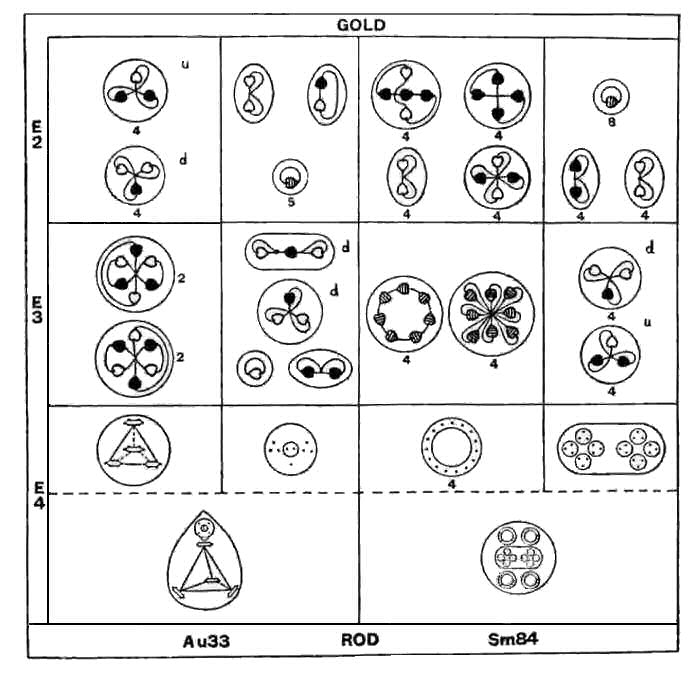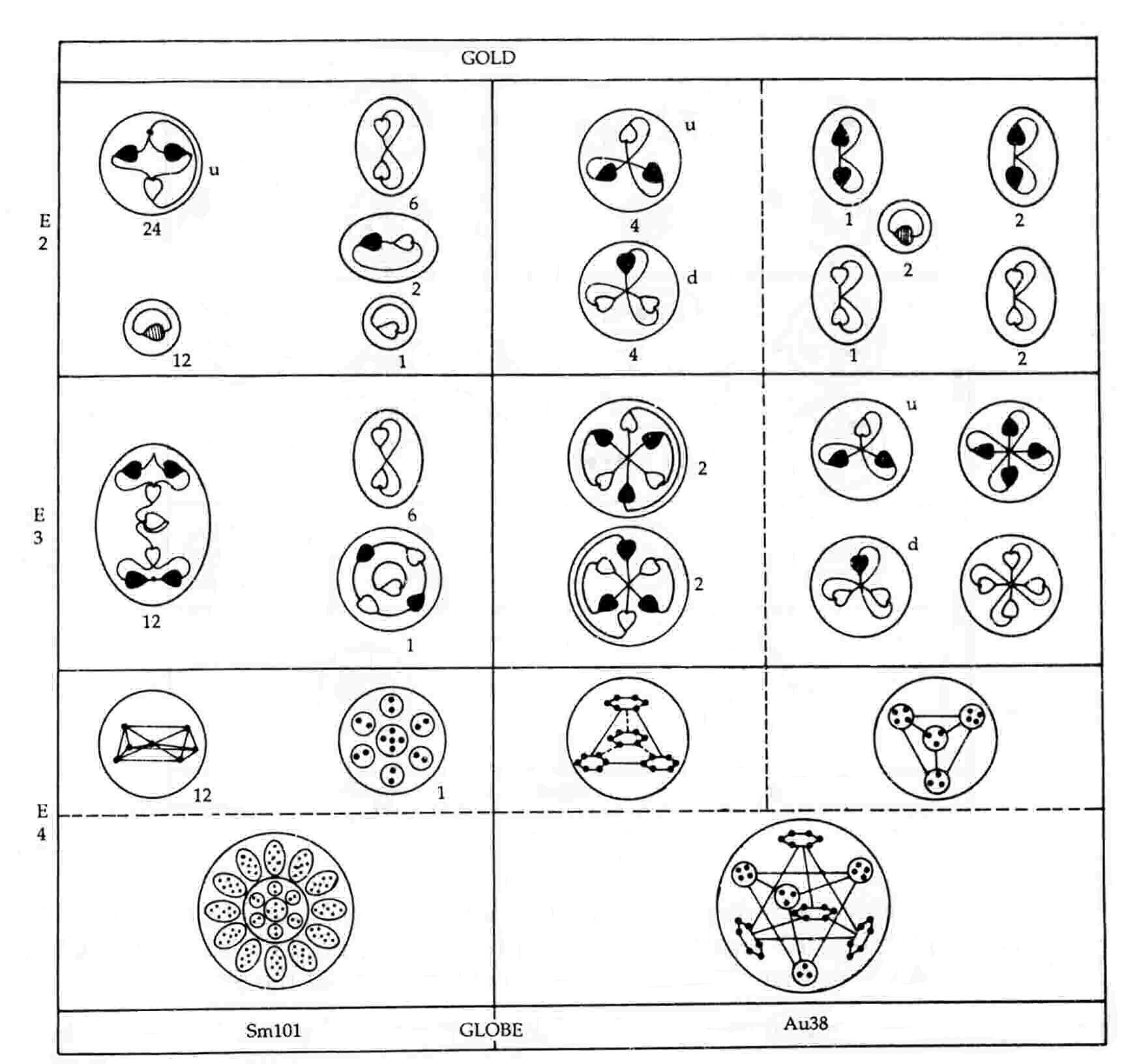Disintegration diagram a Disintegration diagram b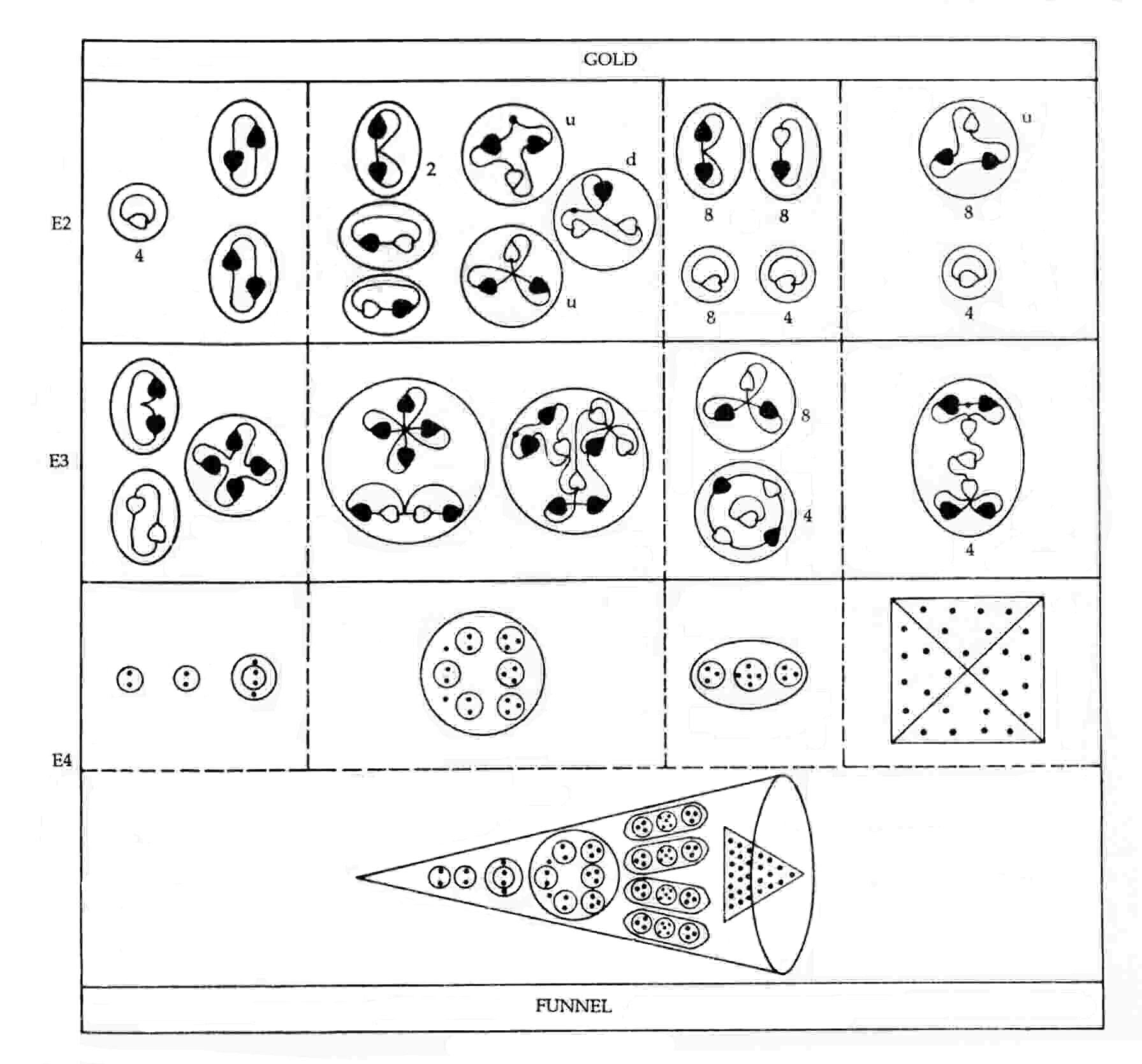Disintegration diagram c

References

1. The Sm84 group is a cluster of bound states of 84 UPAs found in the MPA of samarium.

2. ESP of Quarks and Superstrings, pp. 99-101.

3. Analysis of the Occultum MPA reveals that this ring comprises the nine X subquarks and six Y subquarks making up four u quarks and one d quark. See ref. 2, p. 100.

4. Ibid, pp. 127-128.

5. Ibid, p. 122.

6. Ibid, p. 125.

7. Ibid, p. 215.

Home